# Algebraic independence

(diff) ← Older revision | Latest revision (diff) | Newer revision → (diff)

A concept in the theory of field extensions (cf. Extension of a field). Letbe some extension of a field. The elementsare called algebraically independent overif for each polynomial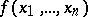with coefficients fromwhich is not identically equal to zero,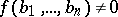. Otherwise, the elementsare called algebraically dependent. An infinite set of elements is called algebraically independent if each one of its finite subsets is algebraically independent; otherwise it is called algebraically dependent. The definition of algebraic independence may be extended to the case whereis a ring, anda subring .

## Algebraic independence of numbers.

Complex numbers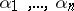are called algebraically independent if they are algebraically independent over the field of algebraic numbers, i.e. if for any polynomial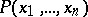with algebraic coefficients, not all of which are zero, the relationship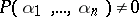is valid. Otherwise,are called algebraically dependent. The concept of algebraic independence of numbers is a generalization of the concept of transcendency of a number (the case). If several numbers are algebraically independent, each one of them is transcendental. It is usually very difficult to prove that given numbers are algebraically independent. The existing analytic methods of the theory of transcendental numbers give a solution of this problem for the values of certain classes of analytic functions. For instance, it was found that the values of the exponential function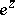, the arguments of which are algebraic and linearly independent over the field of rational numbers, are algebraically independent. A similar result was obtained for Bessel functions (cf. Siegel method). Several general theorems were also established concerning algebraic independence of the values in algebraic points of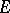-functions which satisfy linear differential equations with coefficients from the field of rational functions , . Algebraic independence has been proved for numbers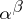and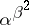, where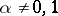is an algebraic number andis a cubic irrationality. A number of theorems have also been proved which deal with the algebraic non-expressibility of numbers; a concept close to that of algebraic independence.

It is possible to impart a quantitative facet to the qualitative concept of algebraic independence if the measure of algebraic independence (cf. Algebraic independence, measure of) of these numbers is considered. The analytic methods referred to above yield estimates from below for the measure of algebraic independence of certain classes of numbers. General theorems treating the estimation of the measure of algebraic independence of the values of-functions have been established .

How to Cite This Entry:
Algebraic independence. Encyclopedia of Mathematics. URL: http://encyclopediaofmath.org/index.php?title=Algebraic_independence&oldid=11559
This article was adapted from an original article by A.B. Shidlovskii (originator), which appeared in Encyclopedia of Mathematics - ISBN 1402006098. See original article